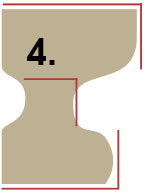Products
Products
topsoil sale
Required Field Label Form FieldOrder Bulk TOPSOIL Below

*
*
*Contact me to order:
*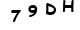## Calculation Help

First, all units of measure must be converted to feet. If you are measuring in both feet and inches, convert the inches using this formula:
Inches ÷ 12 (Example: 6 inches ÷ 12 = 0.5 feet)

### Calculate Cubic Yards Of Topsoil To Order

STEP 1:

Measure length and width of area to be covered and multiply these numbers together.
TIP: See examples below if you have an irregular area to measure before proceeding to STEP 2.
Length x Width (Example: 20 ft x 10 ft = 200 square feet)

STEP 2:

Multiply your previous answer with the thickness (depth) of soil you want added. Since this is often in inches, you will have to use the conversion to feet shown above. So 10 inches = 0.833 feet.
Previous Answer x Depth (200 sq ft x 0.8333 ft = 166.66 cubic feet)

STEP 3:

Convert cubic feet answer shown above to cubic yards
Previous Answer ÷ 27 (Example 166.66 cu ft ÷ 27 = 6.2 cubic yards to order)

### TIP: Calculating Irregular Areas

If your area is not square use the following guidelines to complete STEP 1 above. These may not offer perfect calculations but will give you a more accurate cubic yard measurement when ordering topsoil.

 Square Area Calculation This is the standard method shown in STEP 1 above. Length x Width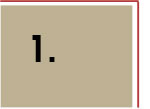Curved Side Area Move your measurement left or right along the curve to balance the area to be covered with the area that is not to be covered. If this is still too difficult to judge, follow guideline for Odd Shapes shown below. Length x Width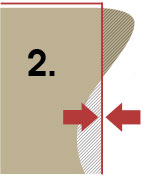Joined Shapes Areas Visually divide the area to be covered into parts for easier measurement. As shown here, dividing this area into three parts makes things easier. In this case, measure the two rectangles and one triangle, then add them together. Length x Width for top rectangle area PLUS Length x Width for bottom rectangle area PLUS Length(b.) x Width(a.) ÷ 2 for the triangle area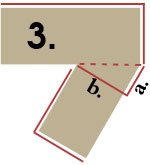Odd Shape Areas Try to find square shapes within an odd shape and add them together. In this example, there are three squares found in an odd shape. Length x Width for top rectangle area PLUS Length x Width for center rectangle area PLUS Length x Width for bottom rectangle area